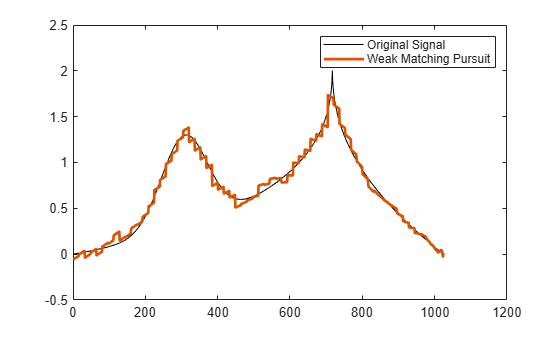# matchingPursuit

Recover sparse signal using matching pursuit algorithm

## Syntax

``[Xr,YI,I,R] = matchingPursuit(A,Y)``
``[Xr,YI,I,R] = matchingPursuit(___,Name=Value)``

## Description

example

````[Xr,YI,I,R] = matchingPursuit(A,Y)` recovers the sparse signal `Xr` using the `sensingDictionary` `A` and sensor measurement `Y`. By default, the sparse recovery algorithm is matching pursuit. The `I` output is the support of `Xr` identified by the matching pursuit algorithm. The `YI` output is the best fit for `Y` corresponding to the bases indexed by the elements of `I`, and `R` is the residual.```
````[Xr,YI,I,R] = matchingPursuit(___,Name=Value)` specifies options using one or more name-value arguments in addition to the input argument in the previous syntax. For example, ```[Xr,YI,I,R] = matchingPursuit(A,Y,Algorithm="WMP")``` specifies the weak matching pursuit algorithm.```

## Examples

collapse all

`load cuspamax`

Create a sensing dictionary consisting of the basis types `poly` and `walsh` that can be applied to the signal.

```lsig = length(cuspamax); A = sensingDictionary(Size=lsig,Type={'poly','walsh'})```
```A = sensingDictionary with properties: Type: {'poly' 'walsh'} Name: {'' ''} Level: [0 0] CustomDictionary: [] Size: [1024 2048] ```

Use the dictionary to obtain a sparse approximation of the signal using weak matching pursuit.

```[Xr,YI,I,R] = matchingPursuit(A,cuspamax, ... Algorithm="WMP",maxerr={"L2",1});```

Plot the original signal and the approximation.

```plot(cuspamax,"k") hold on plot(YI,LineWidth=2) legend("Original Signal","Weak Matching Pursuit") hold off```Extract the vectors from the dictionary that correspond to the approximation. Multiply with the associated coefficients and confirm the result is equal to the approximation.

```Amat = subdict(A,1:1024,I); x = Amat*Xr(I,:); max(abs(x-YI))```
```ans = 0 ```

## Input Arguments

collapse all

Sensing dictionary, specified as a `sensingDictionary` object.

Sensor measurements, specified as a vector `Y` such that ```Y = AX```, where `X` is a sparse signal.

Data Types: `single` | `double`
Complex Number Support: Yes

### Name-Value Arguments

Specify optional pairs of arguments as `Name1=Value1,...,NameN=ValueN`, where `Name` is the argument name and `Value` is the corresponding value. Name-value arguments must appear after other arguments, but the order of the pairs does not matter.

Example: `Xr = matchingPursuit(D,Y,Algorithm="WMP")` recovers the sparse signal using weak matching pursuit.

Recovery algorithm, specified as one of these:

• `"BMP"` — Matching pursuit

• `"OMP"` — Orthogonal matching pursuit

• `"WMP"` — Weak matching pursuit

Example: `[Xr,YI,I,R] = matchingPursuit(D,Y,Algorithm="OMP")` recovers the sparse signal using orthogonal matching pursuit.

Optimality factor for weak orthogonal matching pursuit, specified as a scalar in the interval (0,1]. This option is valid only when `Algorithm` is `"WMP"`.

Example: ```[Xr,YI,I,R] = matchingPursuit(D,Y,Algorithm="WMP",wmpcfs=0.7)``` specifies an optimality factor of `0.7`.

Data Types: `single` | `double`

Maximum number of iterations to recover the sparse signal, specified as a positive integer. The pursuit algorithm stops if the number of iterations reaches `maxIterations`. Note that the number of iterations `matchingPursuit ` performs to recover the sparse signal is equal to the length of the index vector `I`.

Example: `[Xr,YI,I,R] = matchingPursuit(D,Y,maxIterations=15)` iterates at most 15 times to recover the sparse signal.

Data Types: `single` | `double`

Maximum error criteria used to recover the sparse signal, specified as cell array `{NORME,ME}`. `NORME` specifies the norm used in the error computation. Valid options are `"L1"`, `"L2"`, and `"Linf"`. `ME` is a positive scalar in the interval (0,100] that specifies the maximum percentage of the relative admissible value.

The relative error expressed as a percentage is

`$100\frac{‖R‖}{‖Y‖}$`

where R is the residual at each iteration and Y is the input signal. For example, `{"L1",10}` sets maximum acceptable ratio of the L1 norms of the residual to the input signal to 0.10.

If you specify `maxerr`, the matching pursuit terminates when the first of the following conditions is satisfied:

• The number of iterations reaches `maxIterations`.

• The relative error falls below the percentage you specify with the `maxerr` name-value argument.

Example: `[Xr,YI,I,R] = matchingPursuit(D,Y,maxerr={"L1",20})` specifies the L1 norm and a relative error of 20%.

Data Types: `cell`

## Output Arguments

collapse all

Sparse signal recovered, returned as a vector.

Data Types: `single` | `double`
Complex Number Support: Yes

Best fit to the sensor measurements, returned as a vector. `YI` is the best fit for `Y` corresponding to the bases indexed by the elements of `I`. The best fit is defined as ```YI = A(:,I)*Xr(I,:)```.

Data Types: `single` | `double`
Complex Number Support: Yes

Index of basis elements identified by the matching pursuit algorithm, returned as a vector. For matching pursuit algorithms, the length of `I` corresponds to the number of iterations the algorithm needed before termination.

Data Types: `double`

Residual, returned as a vector. The vectors `R` and `Y` are equal in size. The residual is defined as ```R = Y-(A(:,I)*Xr(I,:)) = Y-YI```.

Data Types: `single` | `double`
Complex Number Support: Yes

## Version History

Introduced in R2022a